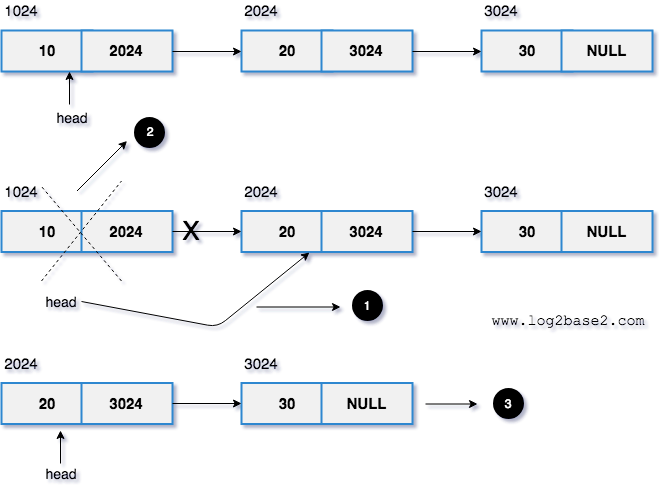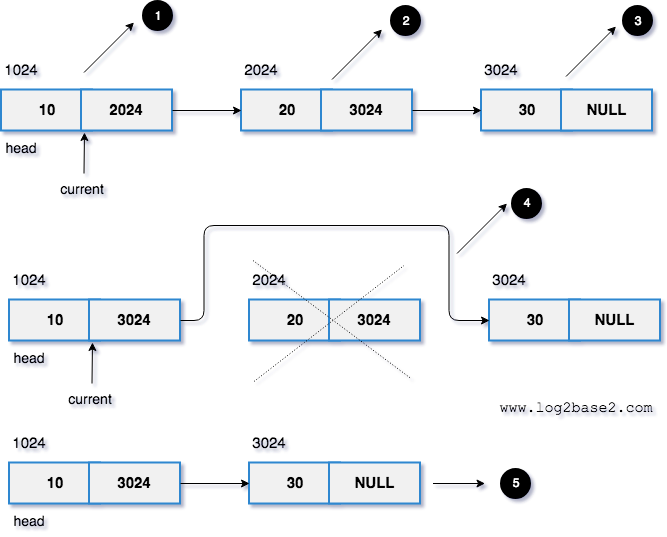# Deleting a node in linked list

Delete a given node from the linked list.

## Example

Linked List : 10 20 30 NULL

20

10 30 NULL

30

10 20 NULL

## Algorithm

1. If the head node has the given key,

make the head node points to the second node and free its memory.

2. Otherwise,

From the current node, check whether the next node has the given key

if yes, make the current->next = current->next->next and free the memory.

else, update the current node to the next and do the above process (from step 2) till the last node.

## 1. Head node has the given key

```void deleteNode(struct node **head, int key)
{
//temp is used to freeing the memory
struct node *temp;

//key found on the head node.
{
free(temp);
}

}
```

#### Visual Representation

Let's delete data 10 (head node).1. Make the head points to the next node.

2. Free the head node's memory.

3. Finally, the new linked list.

## 2. For other nodes (Non-Head)

```void deleteNode(struct node **head, int key)
{
//temp is used to freeing the memory
struct node *temp;

//key found on the head node.
{
temp = *head;    //backup to free the memory
free(temp);
}

else
{
while(current->next != NULL)
{
//if yes, we need to delete the current->next node
if(current->next->data == key)
{
temp = current->next;
//node will be disconnected from the linked list.
current->next = current->next->next;
free(temp);
break;
}
//Otherwise, move the current node and proceed
else
current = current->next;
}
}

}
```

#### Visual Representation

Let's delete data 20.1. Make the current node points to the head node. (current => data = 10).

2. current => next. (current=>next=>data = 20).

3. current => next => next. (current=>next=>next=>data = 30).

4. We have to remove the node 20. Since current => next = 20 we can remove the node by setting current => next = current =>next => next. And then free the node.

5. Finally, the new linked list.

## Implementation of deleting a node in linked list

Example

```/*
* Program: Deleting a node in the linked list
* Language: C
*/

#include<stdio.h>
#include<stdlib.h>

struct node
{
int data;
struct node *next;
};

{
//create a new node
struct node *newNode = malloc(sizeof(struct node));
newNode->data = val;
newNode->next     = NULL;

//if head is NULL, it is an empty list
//Otherwise, find the last node and add the newNode
else
{

//last node's next address will be NULL.
while(lastNode->next != NULL)
{
lastNode = lastNode->next;
}

lastNode->next = newNode;
}

}

void deleteNode(struct node **head, int key)
{
//temp is used to freeing the memory
struct node *temp;

//key found on the head node.
{
temp = *head;    //backup to free the memory
free(temp);
}
else
{
while(current->next != NULL)
{
//if yes, we need to delete the current->next node
if(current->next->data == key)
{
temp = current->next;
//node will be disconnected from the linked list.
current->next = current->next->next;
free(temp);
break;
}
//Otherwise, move the current node and proceed
else
current = current->next;
}
}
}

{

//iterate the entire linked list and print the data
while(temp != NULL)
{
printf("%d ->", temp->data);
temp = temp->next;
}
printf("NULL\n");
}

int main()
{

//delete first node
printf("Deleted 10. The New Linked List:\n");

//delete last node